### Home > CALC > Chapter 9 > Lesson 9.2.2 > Problem9-64

9-64.
1. Examine the integrals below. Consider the multiple tools available for integrating and use the best strategy. After evaluating each integral, write a short description of your method. Homework Help ✎

1.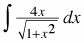2.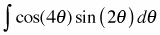3.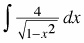4.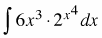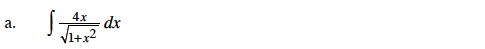Use substitution: Let u = x2 + 1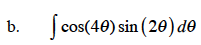cos(4θ) = cos(2(2θ)) = 2(cos2(2θ)) − 1

Substitute using the hint, then integrate using substitution.
Let u = cos(2θ).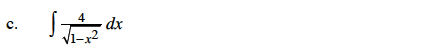The integrand is a multiple of an inverse trigonometric function derivative.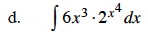Use substitution: Let u = x4